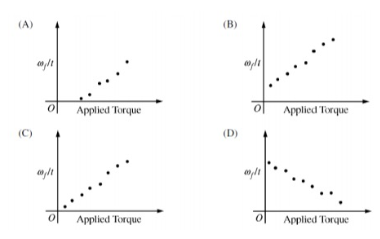# Problem: A student conducts an experiment to determine the relationship between applied torque and the change in angular velocity. The student uses the apparatus shown in the figure above, consisting of two disks that are glued together and mounted on a horizontal axle. Blocks of varying mass are hung from a string would around the smaller disk. The blocks are released from rest, exerting different torques on the disks, and are allowed to fall a fixed distance. For each block, the time of fall t and the final angular velocity are measured. There is considerable friction between the disks and the axle. Which of the following best represents a plot that can be obtained from the student's data?

###### FREE Expert Solution

Torque:

$\overline{){\mathbit{\tau }}{\mathbf{=}}{\mathbit{\alpha }}{\mathbit{I}}}$ where I is the moment of inertia.

α = Δω/Δt = (ωf - ωi)/(t - t0

ωf is the final angular velocity, ωi is the initial angular velocity, t is the final time and t0 is the initial time.

99% (164 ratings)###### Problem Details

A student conducts an experiment to determine the relationship between applied torque and the change in angular velocity. The student uses the apparatus shown in the figure above, consisting of two disks that are glued together and mounted on a horizontal axle. Blocks of varying mass are hung from a string would around the smaller disk. The blocks are released from rest, exerting different torques on the disks, and are allowed to fall a fixed distance. For each block, the time of fall t and the final angular velocity are measured. There is considerable friction between the disks and the axle. Which of the following best represents a plot that can be obtained from the student's data?